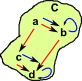# Maths - Hom-Set

In order to treat a function as a type it must hold all possible mappings. An instance of that is an individual mapping.

The hom-set is the set of possible functions/mappings/morphisms between a domain and codomain.

### Hom-Set in Sets

 In sets between a domain set with A elements to a codomain set with X elements denoted A -> X there are XA possible mappings. In the example (diagram right) between a domain set with 2 elements to a codomain set with 3 elements there are 32 = 9 possible mappings.An element of the homset acts on a whole object. So the homset is above the level of functions and objects.

### Hom-Set as a type

In type theory, types can be things such as Integer, Real (Float in computing), Boolean etc. In addition to these types a function can also be a type such as Integer -> Boolean can also be a type. This type is a hom-set, it consists of a set of possible functions such as:

• x < 0
• x² < 16
• and so on ...

As we can se fro the diagram above each element of the hom-set is a function and each of these functions operates on a complete set. So we have a 3-layer hierarchy:

• A complete hom-set.
• An individual function from that hom-set.
• An individual element in the domain which maps to an individual element in the codomain.

So the middle layer relates to an element in the set of functions but the whole 'set' in the domain/codomain.

The equation:

a(b+c) = ab ac

where a,b and c are types, also suggests a way of going between whole sets and elements of a set.

### Hom-Set in Category Theory

If C is a category containing objects A and B then Hom(A,B) is the collection of all morphisms from A to B:

Hom(A,B) = {fC | f: A -> B }

where:

• A = Dom(f)
• B = Cod(f)

## Internal Hom-SetMany types of category can be represented by a graph which can be shown as a set of objects and a set of arrows.

The graph of one (red) endofunctor might be represented by:

• Its objects: {a,b,c,d}
• and its arrows {(a,b),(b,d),(a,c),(c,d)}

and the other (blue) endo functor by

• arrows {(a,b),(b,b),(c,d),(d,d)}

So the set of functors would be:

{{(a,b),(b,d),(a,c),(c,d)},
{(a,b),(b,b),(c,d),(d,d)}}

 we could index this (1:a, 2:b ...) to give: {{(1,2),(2,4),(1,3),(3,4)}, {(1,2),(2,2),(3,4),(4,4)}}In category theory we try to define things using their external properties. So the set of morphisms could be shown externally.

## External Hom-SetHere the morphism goes from object A to object B as suggested by the general definition at the top of the page.Again, in category theory, we try to define things using their external properties. So the set of morphisms could be shown externally.

## Relation to Exponential Object

An internal hom-set in a cartesian closed category is an exponential object.

homC(Z,XY) = homC(Z×Y,X)

## Representable Functor

We can map objects of a category to functors (represented by a homset) like this:

 HA = C(A,-) is a contravarient representable at A HA = C(-,A) is a covarient representable at A

where:

• C(A,-) means B -> C(A,B)
• C(-,A) means B -> C(B,A)

That is, the dash '-' represents what we are mapping from.

## Composing Homsets

Homsets can be composed:

hom(y,z)hom(x,y)->hom(x,z)

## Enriched Categories

So far diagrams on hom-sets are valid upto equality, usually in category theory diagrams are upto isomorphism.

## Programming

The sage program (see this site) can operate on hom-sets like this:

```sage: V = VectorSpace(QQ,3)
sage: Hom(V, V)```

Set of Morphisms from Vector space of dimension 3 over Rational Field to Vector space of dimension 3 over Rational Field in Category of vector spaces over Rational Field

```sage: G = AlternatingGroup(3)
sage: Hom(G, G)```

Set of Morphisms from AlternatingGroup(3) to AlternatingGroup(3) in Category of finite permutation groups

`sage: Hom(ZZ, QQ, Sets())`

Set of Morphisms from Integer Ring to Rational Field in Category of sets

`sage: Hom(FreeModule(ZZ,1), FreeModule(QQ,1))`

Set of Morphisms from Ambient free module of rank 1 over the principal ideal domain Integer Ring to Vector space of dimension 1 over Rational Field in Category of modules with basis over Integer Ring

`sage: Hom(FreeModule(QQ,1), FreeModule(ZZ,1))`

Set of Morphisms from Vector space of dimension 1 over Rational Field to Ambient free module of rank 1 over the principal ideal domain Integer Ring in Category of vector spaces over Rational Field

## Proof

On site here are definitions in Isabella.

Where I can, I have put links to Amazon for books that are relevant to the subject, click on the appropriate country flag to get more details of the book or to buy it from them.The Princeton Companion to Mathematics - This is a big book that attempts to give a wide overview of the whole of mathematics, inevitably there are many things missing, but it gives a good insight into the history, concepts, branches, theorems and wider perspective of mathematics. It is well written and, if you are interested in maths, this is the type of book where you can open a page at random and find something interesting to read. To some extent it can be used as a reference book, although it doesn't have tables of formula for trig functions and so on, but where it is most useful is when you want to read about various topics to find out which topics are interesting and relevant to you.